# JP2004126486A - Device and method for correcting pattern size - Google Patents

## Info

Publication number
JP2004126486A
JP2004126486A JP2002322899A JP2002322899A JP2004126486A JP 2004126486 A JP2004126486 A JP 2004126486A JP 2002322899 A JP2002322899 A JP 2002322899A JP 2002322899 A JP2002322899 A JP 2002322899A JP 2004126486 A JP2004126486 A JP 2004126486A
Authority
JP
Japan
Prior art keywords
pattern
aperture ratio
correction
correcting
test
Prior art date
Legal status (The legal status is an assumption and is not a legal conclusion. Google has not performed a legal analysis and makes no representation as to the accuracy of the status listed.)
Pending
Application number
JP2002322899A
Other languages
Japanese (ja)
Inventor
Hajime Aoyama
Kozo Ogino
Moriyoshi Osawa
Teruyoshi Yao

Original Assignee
Fujitsu Ltd

Priority date (The priority date is an assumption and is not a legal conclusion. Google has not performed a legal analysis and makes no representation as to the accuracy of the date listed.)
Filing date
Publication date
Priority to JP2002224036 priority Critical
Application filed by Fujitsu Ltd, 富士通株式会社 filed Critical Fujitsu Ltd
Priority to JP2002322899A priority patent/JP2004126486A/en
Publication of JP2004126486A publication Critical patent/JP2004126486A/en
Application status is Pending legal-status Critical

• 230000000694 effects Effects 0 abstract 2
• 238000001459 lithography Methods 0 abstract 1

## Images

•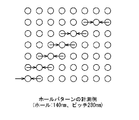•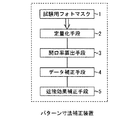•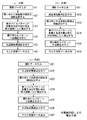•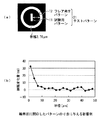•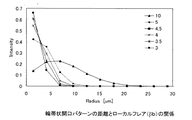•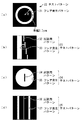•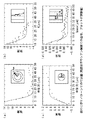•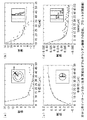•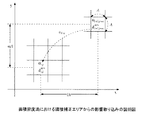•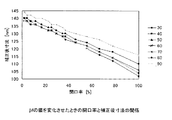•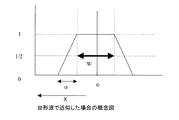•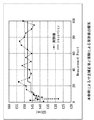•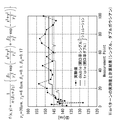•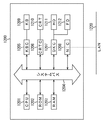## Classifications

• GPHYSICS
• G03PHOTOGRAPHY; CINEMATOGRAPHY; ANALOGOUS TECHNIQUES USING WAVES OTHER THAN OPTICAL WAVES; ELECTROGRAPHY; HOLOGRAPHY
• G03FPHOTOMECHANICAL PRODUCTION OF TEXTURED OR PATTERNED SURFACES, e.g. FOR PRINTING, FOR PROCESSING OF SEMICONDUCTOR DEVICES; MATERIALS THEREFOR; ORIGINALS THEREFOR; APPARATUS SPECIALLY ADAPTED THEREFOR
• G03F1/00Originals for photomechanical production of textured or patterned surfaces, e.g., masks, photo-masks, reticles; Mask blanks or pellicles therefor; Containers specially adapted therefor; Preparation thereof
• G03F1/36Masks having proximity correction features; Preparation thereof, e.g. optical proximity correction [OPC] design processes
• GPHYSICS
• G03PHOTOGRAPHY; CINEMATOGRAPHY; ANALOGOUS TECHNIQUES USING WAVES OTHER THAN OPTICAL WAVES; ELECTROGRAPHY; HOLOGRAPHY
• G03FPHOTOMECHANICAL PRODUCTION OF TEXTURED OR PATTERNED SURFACES, e.g. FOR PRINTING, FOR PROCESSING OF SEMICONDUCTOR DEVICES; MATERIALS THEREFOR; ORIGINALS THEREFOR; APPARATUS SPECIALLY ADAPTED THEREFOR
• G03F1/00Originals for photomechanical production of textured or patterned surfaces, e.g., masks, photo-masks, reticles; Mask blanks or pellicles therefor; Containers specially adapted therefor; Preparation thereof
• G03F1/38Masks having auxiliary features, e.g. special coatings or marks for alignment or testing; Preparation thereof
• G03F1/44Testing or measuring features, e.g. grid patterns, focus monitors, sawtooth scales or notched scales

## Abstract

<P>PROBLEM TO BE SOLVED: To quantitatively estimating size variation in a pattern which is exposed in lithography and to easily and accurately correct the pattern size based upon that. <P>SOLUTION: The correcting device comprises a photomask 1 for test which has a test pattern, a quantitating means 2 which uses the photomask 1 for test to perform quantitation based upon of the relation of the size variation of the test pattern as a function of distance with aperture ratio, an aperture ratio calculating means 3 which divides an exposure area that the test pattern has into a plurality of correction areas and calculates aperture ratios by the correction areas, a data correcting means 4 which substitutes the aperture ratios calculated by the aperture ratio calculating means 3 in the result of the quantitation using the photomask 1 for test to calculate size variations of a real device pattern by the correction areas and then corrects design data of the actual device pattern according to the size variations, and a proximity effect correcting means 5 which corrects proximity effect. <P>COPYRIGHT: (C)2004,JPO

## Description


TECHNICAL FIELD OF THE INVENTION
The present invention relates to a pattern dimension correction apparatus and a correction method for correcting the dimensions of a device pattern formed in a lithography step, which is one of the manufacturing steps of a semiconductor device, for example, a semiconductor chip such as an IC or LSI, or a display element such as a liquid crystal panel. It is suitable for application to the manufacture of various devices such as a detection element such as a magnetic head and an imaging element such as a CCD.

[Prior art]
2. Description of the Related Art In a pattern forming technique, a semiconductor device is manufactured using optical lithography. Optical lithography is a technique in which a desired pattern is formed on a reticle, which is a photomask, and the pattern is transferred onto a sample substrate via a reduction optical system. A photosensitive resin called a resist is formed on the sample substrate, and the latent image of the transferred pattern is etched by forming a pattern using the difference in the dissolution rate between the exposed and unexposed parts by the developer. Thereby, desired material processing can be performed.

In the exposure technique, in order to transfer a fine pattern with high precision, the wavelength of exposure light, the reticle structure are optimized, and in addition, the influence of a nearby pattern is calculated and calculated for correction. This correction is called OPC (Optical Proximity Correction), and calculates the influence of the optical proximity effect on the transferred image of the pattern by taking into account the illumination conditions (NA, Sigma) and exposure conditions (resist material, exposure wavelength) of the exposure apparatus. A correction amount is calculated by an experiment to correct the reticle size.

However, there is a component that cannot be corrected by the OPC, for example, a dimensional difference of a dense / dense pattern due to a flare of an exposure apparatus or etching, and the size of a formed pattern varies. Flare of the exposure apparatus is caused by minute unevenness of the lens, fluctuation of the refractive index, and reflected and scattered light on the wafer surface. Furthermore, recently, the occurrence of local flare depending on the situation around each pattern has been regarded as a problem. This is so-called local flare, which is caused by the specificity of the lens material depending on the wavelength of the exposure light to be used (short wavelength represented by 193 nm) and the like. It is the main cause of unexpected changes. In addition, in the etching, in a region having a large area to be etched, a lack of a reaction gas or an increase in a reaction product occurs, and the pattern formation accuracy is deteriorated due to the area dependency and the size dependency in an arbitrary region.

[Patent Document 1]
JP 2001-52999 A
[Patent Document 2]
JP 2001-112787 A
[Patent Document 3]
JP 2001-153233 A
[Non-patent document 1]
Tae Moon Jong, and soon (Samsung), 'Flare in Microlithographic Exposure Tools', Jpn. J. Appl. Phys. Vol. 41 (2002) 5113.

[Problems to be solved by the invention]
However, it is difficult to accurately correct a dimensional change due to a local flare of the exposure apparatus or a loading effect in etching.

The local flare described above must be quantified and removed in order to form a desired pattern in a semiconductor device in a favorable manner. Therefore, at present, there is no suitable method for consciously solving this problem by specifically identifying this local flare.

In the case of the optical proximity effect correction, the optical proximity effect is calculated from a pattern in a region of several μm. However, it is difficult to calculate the optical proximity effect to a range of several tens of μm due to flare due to time constraints. Yes, and it was difficult to explain by the proximity effect of light intensity. In addition, the effect of the loading effect on the etching cannot be naturally explained by the light intensity calculation.

The present invention has been made to solve the above problem, and quantitatively estimates a dimensional variation occurring in a pattern to be exposed in lithography, and easily and accurately corrects a pattern dimension based on the quantitative variation to achieve extremely high reliability. It is an object of the present invention to provide a pattern dimension correction apparatus and a correction method that can manufacture a device having high accuracy.

[Means for Solving the Problems]
As a result of intensive studies, the present inventor has reached various aspects of the invention described below.

A pattern dimension correcting apparatus and a pattern correcting method according to the present invention are intended to correct a dimensional change that occurs in an actual device pattern formed in photolithography depending on an aperture ratio of a light transmitting region around the actual device pattern.

The pattern dimension correcting apparatus of the present invention uses a test photomask having a test pattern and the test photomask to quantify the dimensional variation in the test pattern as a function of distance in relation to the aperture ratio. Quantification means, an exposure area having a plurality of the actual device patterns, divided into a plurality of correction areas, and an aperture ratio calculation means for calculating the aperture ratio for each of the correction areas; Data correction means for inputting the aperture ratio into the result of the quantification, calculating the dimensional variation of the actual device pattern for each of the correction regions, and correcting the design data of the actual device pattern based on the calculated data. .

Another aspect of the pattern dimension correcting apparatus of the present invention is an aperture ratio calculating unit that divides an exposure region having a plurality of actual device patterns into a plurality of correction regions, and calculates an aperture ratio of a light transmission region for each of the correction regions. And a data correction means for calculating a dimensional change occurring in the real device pattern for each correction area using the calculated aperture ratio, and correcting design data of the real device pattern based on the calculated dimensional change.

The pattern dimension correcting method according to the present invention includes a first step of quantifying the dimension variation in the test pattern as a function of a distance in relation to the aperture ratio using a test photomask having a test pattern; Dividing the exposure area having the actual device pattern into a plurality of correction areas, calculating the aperture ratio for each correction area, and inputting the calculated aperture ratio to the result of the quantification. Calculating the dimensional variation of the actual device pattern for each of the correction areas, and correcting the design data of the actual device pattern based on the third step.

Another aspect of the pattern dimension correction method of the present invention is a first step of dividing an exposure region having a plurality of actual device patterns into a plurality of correction regions and calculating an aperture ratio of a light transmission region for each of the correction regions. And a second step of calculating a dimensional change occurring in the actual device pattern for each correction area using the calculated aperture ratio, and correcting the design data of the actual device pattern based on the calculated dimension variation.

BEST MODE FOR CARRYING OUT THE INVENTION
-Basic gist of the present invention-
First, the basic gist of the present invention will be described.
The present inventors have found that the influence of the loading effect on local flare or etching that causes dimensional variation in a pattern occurs in relation to the aperture ratio of a pattern close to a pattern of interest and the distance between the patterns.

For example, when a hole pattern as shown in FIG. 1 is exposed and developed by an ArF exposure apparatus, when measured from the periphery toward the center, the hole size gradually changes by 10 nm or more over several tens μm from the periphery. On the other hand, when the light proximity effect simulation is performed on the hole pattern, only the dimension of the outermost pattern is reduced by about 2 nm. That is, it is understood that a difference between the result of the experiment and the simulation of the optical proximity effect has occurred, and that the pattern dimension varies with the distance and the aperture ratio, and that the influence of local flare has occurred.

That is, in an arbitrary actual device pattern of interest (a device pattern actually produced in a lithography process), a pattern around the actual device pattern is searched for, and the aperture ratio of the actual device pattern is calculated as a function of distance. The function needs to be able to strongly express the influence of a closer place, and a Gaussian function, a double Gaussian function, a triangular wave function, or the like is appropriate. At this time, in the present invention, the exposure area having each actual device pattern is divided into a plurality of correction areas, and the aperture ratio is calculated for each correction area in consideration of an improvement in processing speed. Specifically, the exposure area is divided into mesh correction areas on the order of several μm on each side, and the calculation is performed assuming that the aperture ratio is uniform within the mesh. This is called an area density method, and can greatly improve the processing speed.

To calculate the dimensional variation of the actual device pattern, the light intensity profile is approximated by a Gaussian or trapezoidal function, and the correlation between the aperture ratio calculated from the pattern data and the actual dimension of the pattern formed on the sample substrate measured by SEM or the like. Ask for. Thus, the aperture ratio around the arbitrary pattern can be approximated by the function, and the dimensional change can be predicted. By correcting the design data of the actual device pattern, a photomask (reticle) having a pattern in which the influence of local flare in optical lithography has been corrected can be manufactured. When the reticle size is corrected, since there is an optical proximity effect, the dimensional change is converted into a reticle dimensional change amount or an optical proximity effect correction process is performed to create desired reticle dimension data.

-Specific embodiment-
Hereinafter, specific embodiments will be described based on the basic gist of the present invention described above.
In the present embodiment, a pattern dimension correction apparatus and a correction method for correcting a dimension of a device pattern formed in a lithography process are disclosed. In the present embodiment, a case is described in which the variation in the pattern dimension is caused by the local flare of the exposure apparatus. However, the effect of the loading effect in the etching process can be similarly derived from the relationship with the aperture ratio of the peripheral pattern. it can.

As shown in FIG. 2, this pattern dimension correcting apparatus uses a test photomask 1 having a test pattern, and uses the test photomask 1 to determine the relationship between the dimensional variation in the test pattern and the aperture ratio as a function of distance. Quantification means 2 for quantifying the exposure area, an exposure area having a plurality of actual device patterns, divided into a plurality of correction areas, and an aperture ratio calculation means 3 for calculating an aperture ratio for each correction area. The calculated aperture ratio is input to the result of the quantification using the test photomask 1, and the dimensional variation of the actual device pattern is calculated for each correction region, and the design data of the actual device pattern is corrected based on the calculated data. It comprises a correction means 4 and a proximity effect correction means 5 for correcting the proximity effect.

Hereinafter, a correction method using the pattern dimension correction device having the above configuration will be described together with the functions of the above-described units.
FIG. 3 is a flowchart showing each procedure of the pattern dimension correction.
In the procedure 1 of FIG. 3A, the dimensions of the actual device pattern are corrected from the aperture ratio of each correction region through steps S2 to S5 described below based on the relationship between the aperture ratio and the pattern dimension variation. The optical proximity effect correction in step S6 using the proximity effect correction means 5 is performed to create design data for the reticle to be manufactured.

In the procedure 2 of FIG. 3B, after the optical proximity effect correction is performed in step S12, the dimensions of the actual device pattern are corrected from the aperture ratio of each correction area through steps S13 to S16 described below. Then, design data for a reticle to be manufactured is created. Since the correction value is a numerical value on the wafer, the dimensional correction value on the reticle is a value corresponding to the exposure condition and the pattern type. As in Procedures 1 and 2, the optical proximity correction is performed incidentally because the optical proximity correction and the dimensional change due to the change in the aperture ratio occur independently, and each correction is performed independently. This is because it is necessary.

Further, in the procedure 3 in FIG. 3C, after performing the optical proximity effect correction in step S22, the optical proximity effect correction is performed in step S27 again through steps S23 to S26 described below, and the reticle is manufactured. Create design data. For example, if the correction based on the pattern aperture ratio is performed after the optical proximity effect correction, the optical proximity effect correction needs to be performed again to form a pattern on the photoresist on the sample substrate according to the corrected design data. This is because

Hereinafter, steps S1 to S6 will be described with reference to FIG.
First, design data is input to the pattern dimension correcting device (step S1).
Subsequently, the quantification unit 2 uses the test photomask 1 to quantify the dimensional variation in the test pattern as a function of distance in relation to the aperture ratio (step S2).

Here, as shown in FIG. 4A, the test photomask 1 has a test pattern 21. Each test pattern has, for example, a test pattern 11 having a pattern dimension (here, line width) of 120 μm. And a flare generation pattern 12 having a width of, for example, 2.76 μm, which forms a ring-shaped light transmission region surrounding the test pattern 11. Here, the relationship between the aperture ratio and the distance given to the test pattern 11 at the center to be measured is obtained by changing the radius of the flare generation pattern 12 for exposure. Exposure is based on exposure of only the test pattern 11 in which an exposure of the flare generation pattern 12 is not performed using a positive resist in an ArF exposure apparatus.

FIG. 4B shows the relationship between the radius of exposure of the flare generation pattern 12 and the pattern dimension variation generated in the test pattern 11. Here, the pattern dimension variation is represented by a difference from the case where exposure is performed using only the reference test pattern 11.
In the region where the radius of exposure of the flare generation pattern 12 is large, it is affected by local flare from a distance. When a certain distance is left, no dimensional fluctuation occurs, and only the reference test pattern 11 is formed. Is obtained (the difference value is a value close to 0). On the other hand, when the flare occurrence pattern 12 approaches, it is found that the measurement value (difference value) of the pattern size of the test pattern 11 increases due to the influence of local flare.

Subsequently, the aperture ratio is calculated by the aperture ratio calculation means 3 for each of the divided correction regions (step S3).
Here, in an arbitrary actual device pattern, the aperture ratio affected by local flare can be calculated by integrating an adjacent pattern as a function of distance in each arbitrary pattern. As a function for approximating the aperture ratio, when γ is the effect of the aperture ratio, a Gaussian function represented by Expression (1) or a triangular wave represented by Expression (2) can be used.

(Equation 1)

As described above, when calculating for any arbitrary pattern, there is a significant delay in processing speed. Therefore, in the present embodiment, in order to obtain a practical processing speed, the exposure region having a plurality of actual device patterns is divided into a plurality of correction regions, here, mesh division is performed using an arbitrary grid, and the aperture ratio is obtained in units of the correction region. ing. Such a method of dividing into correction regions and approximating the pattern density is called an area density method when calculating the influence of backscattered electrons in the electron beam exposure technique (Japanese Patent Application Laid-Open No. 2001-52999). When expressing the pattern aperture ratio in the divided correction area, it is desirable that the size of the divided grid is set to be about 1/10 or less of the range where local flare or the like affects.

For example, FIG. 5 shows the result of calculating the influence (γ) of local flare by the Gaussian function shown in the equation (1) based on the test pattern shown in FIG.
Comparing the experimental result of FIG. 4B with the calculation result of FIG. 5, it can be seen that the γ value is about 5 μm, and the tendency of the distribution coincides. That is, it is considered appropriate that the effect of the aperture ratio is about 5 μm and the correction area is formed by dividing the grid by a grid of about 0.5 μm.

Here, as a function for approximating the aperture ratio, two Gaussian functions (double Gaussian function) or three or more Gaussian functions may be used instead of the Gaussian function (single Gaussian function) shown in equation (1). Can be Thus, taking the double Gaussian function as an example, the applicability of the function is considered.

As the test pattern for the occurrence of local flare, not only the example of FIG. 4A but also the use of, for example, four types as shown in FIGS. 6A to 6D can be considered. FIG. 6A shows a test pattern 22 having only a flare generation pattern 31 having a width of, for example, 2.7 μm which forms a ring-shaped light transmission region, and FIG. 6B shows a band-like flare generation pattern 33 and the flare generation pattern. A test pattern 23 having a plurality of linear test patterns 32 sequentially separated from the test pattern 33, FIG. 6C shows a flare generating pattern 35 penetrated in a disk shape and the flare generating pattern 35. FIG. 6D illustrates a test pattern 24 having a line-shaped light-shielding pattern 34, and FIG. 6D illustrates a test pattern 25 having a band-shaped test pattern 36 and a band-shaped (1 μm wide) flare generation pattern 37 formed in parallel with the test pattern 36. ing.

When calculating the aperture ratio affecting an arbitrary pattern as a function of the distance from the arbitrary pattern, it is possible to infer with four types of patterns as shown in FIGS. The solution of can be derived.

FIG. 7 shows the result of fitting the effect of the aperture ratio with a single Gaussian function. Here, the case of the test pattern of FIG. 6A corresponds to FIG. 7A, FIG. 6B is FIG. 7B, FIG. 6C is FIG. 7C, FIG. FIG. 7D corresponds to FIG. It can be seen that the fitting is different depending on the test pattern. It can be seen that the tendency is that the dimension is changed near an arbitrary pattern while being affected by a greater distance. In other words, when trying to express the experimental result more faithfully, there is a possibility that a plurality of Gaussian functions can be used for more accurate matching.

FIG. 8 shows the result of fitting the effect of the aperture ratio with two Gaussian functions, that is, the following double Gaussian function (1 ′). Here, β is a coefficient when the influence of the aperture ratio is taken into dimensional variation. 8, similarly to FIG. 7, the test pattern of FIG. 6A corresponds to FIG. 8A, FIG. 6B shows FIG. 8B, and FIG. c) and FIG. 6D correspond to FIG. 8D, respectively.

(Equation 2)

As described above, it can be seen that the use of the double Gaussian function is more accurate than the single Gaussian function for all four test patterns.

With reference to FIG. 9, a method of calculating an effective area density based on the influence of local flare by the Gaussian approximation by the area density method will be described. FIG. 9 is a diagram showing a state in which the exposure data is divided into correction areas of a rectangular shape having a size of A × A. Here, since the dimensional variation of the (i + 1, j + m) th correction area separated by (1A, mA) has a spread due to local flare or the like due to the exposure apparatus, the (i, j) th It affects the dimensional fluctuation of the correction area. Contribution a due to local flare from the (i + 1, j + m) -th correction area separated by (1A, mA) l, m Can be expressed by the following equation (3) in a form integrating the influence from the A × A region. In the case of approximation by a triangular wave, the exp part of Expression (3) may be replaced with Expression (2).

[Equation 3]

Here, the area density of the pattern in the (i + 1, j + m) -th correction region separated by (1A, mA) is α i + 1, j + m Then, the contribution of the local flare from the (i + 1, j + m) th correction area is a l, m × α i + 1, j + m It becomes. Therefore, the effective area density α ′ of the (i, j) th correction area after the smoothing processing i, j It is sufficient to take in the contribution from each of the correction areas in an area having a radius of about 2γ with respect to the total as a sum and can be expressed by Expression (4).

(Equation 4)

Subsequently, the relationship between the aperture ratio calculated by the data correction means 4 and the pattern dimension variation is shown as a function (step S4).
As an approximation method, a Gaussian function or a trapezoidal waveform can be used. For example, when a dimension variation value is approximated by a Gaussian function, the parameter related to the dimension variation can be represented by Expression (5), where α is a parameter related to the dimension variation.

(Equation 5)

That is, the dimension in an arbitrary pattern is related to the sum of the values obtained by dividing the expression (4), which takes in the aperture ratio, and the expression (5), which describes the dimensional variation, by the area. Assuming that the corrected pattern dimension is W, the sum of the aperture ratio and the dimensional variation can be calculated from a value that is a certain threshold (Threshold) as shown in Expression (6). Here, β is a coefficient when the influence of the aperture ratio is taken into dimensional variation.

(Equation 6)

As described above, when the correction value of the pattern dimension is calculated by the double Gaussian, it is a problem whether the dimension variation can be sufficiently explained by the Gaussian shown in the first item. Therefore, an example in which the above-described double Gaussian is used to estimate the aperture ratio and dimensional variation in the obtained arbitrary pattern will be described. When approximating the parameters of α, β, and γ required in the equations (3) and (5) to the relationship between the aperture ratio and the pattern size shown in FIG. 4B, the α value is intentionally changed. FIG. 10 shows the result of simulation for a pattern having a pattern size of 140 nm. When the value of α becomes larger than 80 nm, the dimensional correction value starts to deviate from the group in which α is 70 nm or less. This indicates that, for an arbitrary pattern size, there is an effective value of α for expressing the aperture ratio in the pattern dimension, and α needs to be に す る or less of the target pattern size. It turns out that there is.

This is presumed to be due to the fact that the relationship between the aperture ratio and the pattern size fluctuates almost linearly, and in Gaussian, the footprint and the vicinity of the peak suddenly deviate from the linear function. Thus, as shown in FIG. 11, it can be seen that a trapezoidal wave can be used as an approximate function. In the case of approximation by a trapezoidal wave, the first term of Expression (6) can be expressed by Expression (7). The dimensional difference when the aperture ratio changes to the maximum from 0 to 100% is α, the arbitrary strength is １ ／, and the dimension is W.
When 0 ≦ x <w / 2−α / 2, f (x) = 1
When w / 2−α / 2 ≦ x ≦ w / 2 + α / 2 f (x) = 0.5 (x−w / 2) / α−1 / 2x> w / 2 + α / 2 f (x) = 0 ... (7)

In this manner, the aperture ratio in an arbitrary actual device pattern is approximated by a Gaussian or a triangular wave for each correction region, and the variation of the pattern dimension is approximated by a Gaussian or a trapezoidal wave so as to be able to be corrected in relation to the aperture ratio. The procedure is to extract and correct the amount. As a reference, in the electron beam lithography technology, there is a method of approximating forward scattering and back scattering, each of which has a physical meaning, by Gaussian, and performing proximity effect correction for each correction region. 2001-153233).

FIG. 12 shows an evaluation result when an arbitrary pattern dimension is approximated by a Gaussian function based on an area density method and an amount of dimensional change is approximated by a Gaussian function.
In this calculation, the parameters α, β, and γ were fitted from the exposure results shown in FIG. The measured values are values measured by SEM using an ArF exposure apparatus and a positive resist. The evaluation is performed using the hole pattern shown in FIG. 1 (a pattern in which patterns having a pitch of 280 nm and a Via of 140 nm are arranged), and actual measurement and calculation are performed by the SEM from the corner to the center of the pattern region. The horizontal axis is the number measured from the corner to the center of the pattern area, and the vertical axis is the measured and calculated hole size. Although the hole size increases toward the center, it can be understood that the hole size can be explained by a value calculated by the method of the present embodiment. In this case, the variation of the pattern dimension is approximated by Gaussian, but a similar result is obtained with a trapezoidal wave. That is, according to the present embodiment, it is possible to explain that the dimensional fluctuation caused by the influence of the local flare changes according to the aperture ratio, and by correcting the pattern dimensional value based on this value, it is possible to obtain high-precision dimensional accuracy. You can see that.

Further, FIG. 13 shows an evaluation result obtained when an arbitrary pattern dimension is approximated by a double Gaussian function based on the area density method and an amount of dimensional change is approximated by a Gaussian function based on the area density method. .
As described above, when the effect of the aperture ratio is approximated by the double Gaussian function, similarly to the case where the effect is approximated by the single double Gaussian function, it can be explained that the dimensional fluctuation due to the influence of the local flare changes with the aperture ratio. It can be seen that a high dimensional accuracy can be obtained by correcting the pattern dimensional value based on this value.

In the present embodiment, the dimensional correction accompanying the variation in the aperture ratio has been described. However, since the optical proximity effect is exerted in the photolithography as described above, the dimensional correction based on the aperture ratio is performed, and then the optical proximity effect correction is performed. Is added (step S5). Incidentally, in the test pattern 21 of the test photomask 1 in this embodiment, the optical proximity correction amount is such that the mask size is increased by about 2 nm only in the outermost peripheral pattern.

Then, design data of an actual device pattern for producing a reticle is output (step S6).

In the present embodiment, the correction of the influence of the exposure, that is, the influence of the local flare has been described. However, the correction of the loading effect in the etching in which the dimension varies in relation to the aperture ratio can be similarly performed.

As described above, according to the present embodiment, a dimensional variation occurring in a pattern exposed in optical lithography is quantitatively estimated, and the pattern dimension is easily and accurately corrected based on the quantitative variation. The device can be manufactured.

Each unit of the pattern dimension correcting apparatus and method according to the above-described embodiment, and each step of the turn dimension correcting method (steps 1 to 6 in FIG. 3A, steps 11 to 16 in FIG. 3B, FIG. Steps 21 to 27 of (c) can be realized by operating a program stored in a RAM or a ROM of the computer. This program and a computer-readable storage medium storing the program are included in the present invention.

Specifically, the program is recorded on a recording medium such as a CD-ROM or provided to a computer via various transmission media. As a recording medium for recording the program, a flexible disk, a hard disk, a magnetic tape, a magneto-optical disk, a nonvolatile memory card, and the like can be used in addition to the CD-ROM. On the other hand, as a transmission medium of the program, a communication medium (wired line such as an optical fiber or the like) in a computer network (LAN, WAN such as the Internet, wireless communication network, etc.) system for transmitting and supplying program information as a carrier wave is provided. Wireless line, etc.).

In addition, not only the functions of the above-described embodiments are realized by the computer executing the supplied program, but the program cooperates with an OS (operating system) or other application software running on the computer. In the case where the functions of the above-described embodiment are realized, or in the case where all or a part of the processing of the supplied program is performed by a function expansion board or a function expansion unit of a computer to realize the functions of the above-described embodiment. Such a program is also included in the present invention.

For example, FIG. 14 is a schematic diagram illustrating an internal configuration of a general personal user terminal device. In FIG. 14, reference numeral 1200 denotes a computer PC. The PC 1200 includes a CPU 1201, executes device control software stored in a ROM 1202 or a hard disk (HD) 1211 or supplied from a flexible disk drive (FD) 1212, and comprehensively controls each device connected to a system bus 1204. To control.

Hereinafter, various aspects of the present invention will be collectively described as supplementary notes.

(Supplementary Note 1) A pattern dimension correcting apparatus for correcting a dimension variation occurring in an actual device pattern formed in photolithography depending on an aperture ratio of a light transmission region existing around the pattern,
A test photomask having a test pattern;
Using the test photomask, quantification means for quantifying the dimensional variation in the test pattern in relation to the aperture ratio as a function of distance,
An aperture ratio calculation unit that divides an exposure region having a plurality of actual device patterns into a plurality of correction regions, and calculates the aperture ratio for each of the correction regions.
The aperture ratio calculated by the aperture ratio calculation means is input to the result of the quantification, the dimensional variation of the actual device pattern is calculated for each of the correction regions, and the design data of the actual device pattern is Data correction means for correcting
A pattern dimension correcting device characterized by including:

(Supplementary Note 2) The test pattern of the test photomask is a measurement pattern for which the dimensional variation is to be estimated, and a light transmitting region that causes the dimensional variation in the measurement pattern. And a plurality of flare generation patterns having different distances from each other.

(Supplementary Note 3) The quantification means quantifies the dimension of the measurement pattern corresponding to each of the flare occurrence patterns in a relationship between the measurement pattern and the distance between each of the flare occurrence patterns. 3. The pattern dimension correcting apparatus according to claim 2, wherein the dimension variation is quantified based on a relationship with the aperture ratio.

(Supplementary Note 4) The liquid crystal display according to any one of Supplementary Notes 1 to 3, wherein the aperture ratio calculation unit calculates an area density of each of the correction regions, and calculates the aperture ratio by an approximate calculation using a Gaussian function. The described pattern dimension correcting device.

(Supplementary note 5) The supplementary note 1 or 2, wherein the aperture ratio calculating means calculates an area density of each of the correction regions, and calculates the aperture ratio by an approximate calculation using at least two Gaussian functions. 2. The pattern dimension correcting device according to claim 1.

(Supplementary note 6) The data correction means according to any one of Supplementary notes 1 to 5, wherein the dimensional change of the actual device pattern due to the change of the aperture ratio is calculated by an approximate calculation using a Gaussian function. 3. The pattern dimension correcting device according to 1.

(Supplementary Note 7) In the Gaussian function used for calculating the dimensional variation by the data correction unit, a value of 1 / e of Gaussian is equal to or less than 1/2 of a minimum pattern width included in the actual device pattern, and 1/2. 7. The pattern dimension correcting device according to claim 6, wherein the numerical value is close to.

(Supplementary Note 8) The data correction unit according to any one of Supplementary notes 1 to 5, wherein the dimensional variation of the actual device pattern due to the variation of the aperture ratio is calculated by an approximate calculation using a trapezoidal function. 3. The pattern dimension correcting device according to 1.

(Supplementary Note 9) The pattern dimension correcting apparatus according to any one of Supplementary Notes 1 to 8, further including an optical proximity effect correction unit.

(Supplementary Note 10) An aperture ratio calculating unit that divides an exposure region having a plurality of actual device patterns into a plurality of correction regions, and calculates an aperture ratio of a light transmission region for each of the correction regions.
Using the calculated aperture ratio, calculate a dimensional change occurring in the actual device pattern for each correction region, and based on the data correction means for correcting the design data of the actual device pattern,
A pattern dimension correcting device characterized by including:

(Supplementary Note 11) The pattern dimension correcting apparatus according to Supplementary Note 10, wherein the aperture ratio calculation unit obtains an area density of each of the correction regions, and calculates the aperture ratio by an approximate calculation using a Gaussian function.

(Supplementary Note 12) The pattern dimension according to Supplementary Note 10, wherein the aperture ratio calculation unit obtains an area density of each of the correction regions, and calculates the aperture ratio by an approximate calculation using at least two Gaussian functions. Correction device.

(Supplementary Note 13) The data correction unit according to any one of Supplementary Notes 10 to 12, wherein the dimensional change of the actual device pattern due to the change in the aperture ratio is calculated by an approximate calculation using a Gaussian function. 3. The pattern dimension correcting device according to 1.

(Supplementary Note 14) In the Gaussian function used for calculating the dimensional variation by the data correction unit, a value of 1 / e of Gaussian is equal to or smaller than 1/2 of a minimum pattern width included in the actual device pattern, and 1/2. 14. The pattern dimension correcting device according to supplementary note 13, wherein the numerical value is close to.

(Supplementary Note 15) The data correction unit according to any one of Supplementary Notes 10 to 12, wherein the dimensional change of the actual device pattern due to the change in the aperture ratio is calculated by an approximate calculation using a trapezoidal function. 3. The pattern dimension correcting device according to 1.

(Supplementary Note 16) The pattern dimension correcting apparatus according to any one of Supplementary Notes 10 to 15, further including an optical proximity effect correcting unit.

(Supplementary Note 17) A pattern dimension correction method for correcting a dimensional change occurring in an actual device pattern formed in photolithography depending on an aperture ratio of a light transmission region existing around the pattern,
A first step of using a test photomask having a test pattern to quantify the dimensional variation in the test pattern as a function of distance in relation to the aperture ratio;
A second step of dividing the exposure region having the plurality of actual device patterns into a plurality of correction regions, and calculating the aperture ratio for each of the correction regions;
A third step of inputting the calculated aperture ratio to the result of the quantification, calculating the dimensional variation of the actual device pattern for each correction region, and correcting the design data of the actual device pattern based on this. Steps and
A pattern dimension correcting method comprising:

(Supplementary Note 18) The test pattern of the test photomask is a measurement pattern for which the dimensional variation is to be estimated, and a light transmitting region that causes the dimensional variation in the measurement pattern. 18. The pattern dimension correcting method according to claim 17, comprising a plurality of flare occurrence patterns having different distances.

(Supplementary Note 19) In the first step, the dimension of the measurement pattern corresponding to each of the flare occurrence patterns is quantified by a relationship between the measurement pattern and the distance between each of the flare occurrence patterns. 18. The pattern dimension correction method according to claim 18, wherein the dimension variation is quantified based on the relationship with the aperture ratio.

(Supplementary Note 20) The method according to any one of Supplementary Notes 17 to 19, wherein in the second step, an area density of each of the correction regions is obtained, and the aperture ratio is calculated by an approximate calculation using a Gaussian function. The described pattern dimension correction method.

(Supplementary note 21) In the second step, the area density of each of the correction regions is obtained, and the aperture ratio is calculated by an approximate calculation using at least two Gaussian functions. 2. The pattern dimension correction method according to item 1.

(Supplementary note 22) In the third step, the dimensional change of the actual device pattern due to the change in the aperture ratio is calculated by an approximate calculation using a Gaussian function. Pattern dimension correction method described in the paragraph.

(Supplementary Note 23) In the Gaussian function used for calculating the dimensional variation in the third step, the value of 1 / e of Gaussian is less than or equal to 1/2 of the minimum pattern width included in the actual device pattern, and 23. The pattern dimension correcting method according to supplementary note 22, wherein the numerical value is close to 2.

(Supplementary note 24) In the third step, the dimensional variation of the actual device pattern due to the variation of the aperture ratio is calculated by an approximate calculation using a trapezoidal function. Pattern dimension correction method described in the paragraph.

(Supplementary note 25) The pattern dimension correcting method according to any one of Supplementary notes 17 to 24, further comprising a fourth step of performing a means for correcting the optical proximity effect.

(Supplementary Note 26) The fourth step is executed before the first step, after the third step, or both before the first step and after the third step. 25. The pattern dimension correction method according to claim 25, wherein

(Supplementary Note 27) A first step of dividing an exposure region having a plurality of actual device patterns into a plurality of correction regions, and calculating an aperture ratio of a light transmission region for each of the correction regions;
A second step of calculating a dimensional change occurring in the actual device pattern for each correction region using the calculated aperture ratio, and correcting design data of the actual device pattern based on the calculated variation;
A pattern dimension correcting method comprising:

(Supplementary note 28) The pattern dimension correction method according to Supplementary note 27, wherein the first step calculates an area density of each of the correction regions, and calculates the aperture ratio by an approximate calculation using a Gaussian function.

(Supplementary note 29) The pattern dimension according to Supplementary note 27, wherein in the first step, an area density of each of the correction regions is obtained, and the aperture ratio is calculated by an approximate calculation using at least two Gaussian functions. Correction method.

(Supplementary note 30) The second step, wherein the dimensional change of the actual device pattern due to the change in the aperture ratio is calculated by an approximate calculation using a Gaussian function. Pattern dimension correction method described in the paragraph.

(Supplementary Note 31) In the Gaussian function used for calculating the dimensional variation in the second step, the value of 1 / e of Gaussian is less than or equal to 1/2 of the minimum pattern width included in the actual device pattern, and 30. The pattern dimension correcting method according to claim 30, wherein the numerical value is close to 2.

(Supplementary Note 32) In the second step, the dimensional variation of the actual device pattern due to the variation of the aperture ratio is calculated by an approximate calculation using a trapezoidal function. Pattern dimension correction method described in the paragraph.

(Supplementary note 33) The pattern dimension correcting method according to any one of Supplementary notes 27 to 32, further including a third step of performing a correction unit of the optical proximity effect.

(Supplementary Note 34) The third step is executed before the first step or after the second step, or both before the first step and after the second step. 33. The pattern dimension correction method according to attachment 33, wherein

(Supplementary Note 35) When correcting a dimensional change occurring in an actual device pattern formed by photolithography depending on an aperture ratio of a light transmitting region existing around the device pattern,
A first step of using a test photomask having a test pattern to quantify the dimensional variation in the test pattern as a function of distance in relation to the aperture ratio;
A second step of dividing the exposure region having the plurality of actual device patterns into a plurality of correction regions, and calculating the aperture ratio for each of the correction regions;
A third step of inputting the calculated aperture ratio to the result of the quantification, calculating the dimensional variation of the actual device pattern for each correction region, and correcting the design data of the actual device pattern based on this. Steps and
A program for causing a computer to execute.

(Supplementary note 36) A computer-readable recording medium recording the program according to supplementary note 35.

(Supplementary Note 37) a first step of dividing an exposure region having a plurality of actual device patterns into a plurality of correction regions, and calculating an aperture ratio of a light transmission region for each of the correction regions;
A second step of calculating a dimensional change occurring in the actual device pattern for each correction region using the calculated aperture ratio, and correcting design data of the actual device pattern based on the calculated variation;
A program for causing a computer to execute.

(Supplementary note 38) A computer-readable recording medium characterized by recording the program according to supplementary note 37.

【The invention's effect】
According to the present invention, it is possible to quantitatively estimate a dimensional variation occurring in a pattern to be exposed in lithography, easily and accurately correct a pattern dimension based on the quantitative variation, and to manufacture an extremely reliable device. It is possible to provide an apparatus and a method for correcting a pattern dimension.
[Brief description of the drawings]
FIG. 1 is a schematic plan view showing a state when a hole pattern is exposed and developed by an ArF exposure apparatus.
FIG. 2 is a block diagram illustrating a schematic configuration of a pattern dimension correcting apparatus according to the embodiment.
FIG. 3 is a flowchart showing each procedure of pattern dimension correction of the present embodiment.
FIG. 4 is a schematic diagram illustrating a schematic configuration of a test photomask and a relationship between a radius of exposure of an annular pattern and a pattern dimension variation generated in a test pattern.
FIG. 5 is a characteristic diagram showing a result of calculating the influence of local flare using a Gaussian function based on the test pattern shown in FIG.
FIG. 6 is a schematic diagram showing another example of a test pattern for occurrence of local flare.
FIG. 7 is a characteristic diagram showing the result of fitting the effect of the aperture ratio with a single Gaussian function.
FIG. 8 is a characteristic diagram showing the result of fitting the effect of aperture ratio with a double Gaussian function.
FIG. 9 is a schematic diagram for explaining a method of calculating the effective area density based on the influence of local flare by the area density method by Gaussian approximation.
FIG. 10 shows a simulation result of a pattern having a pattern dimension of 140 nm, when parameters of α, β, and γ are approximated to the relationship between the aperture ratio and the pattern dimension, by fitting by changing the α value intentionally. It is a characteristic diagram.
FIG. 11 is a characteristic diagram showing a case where a trapezoidal wave is used as an approximate function.
FIG. 12 is a characteristic diagram showing an evaluation result when an arbitrary pattern dimension is approximated by a Gaussian function based on an area density method and an amount of dimensional change is approximated by a Gaussian.
FIG. 13 is a characteristic diagram in which an evaluation result when an arbitrary pattern dimension is approximated by a double Gaussian function based on an area density method and an amount of dimensional change is approximated by Gaussian is added to FIG. is there.
FIG. 14 is a schematic diagram showing an internal configuration of a general personal user terminal device.
[Explanation of symbols]
2 Quantification means
3 Aperture ratio calculation means
4 Data correction means
5 Proximity effect correction means
11,32,34,36 Test pattern
12,31,33,35,37 Flare generation pattern
21, 22, 23, 24, 25 test pattern

## Claims (10)

1. An actual device pattern formed in photolithography, a pattern size correction device that corrects a size change that occurs depending on the aperture ratio of the light transmission region existing around the device,
A test photomask having a test pattern;
Using the test photomask, quantification means for quantifying the dimensional variation in the test pattern in relation to the aperture ratio as a function of distance,
An aperture ratio calculation unit that divides an exposure region having a plurality of actual device patterns into a plurality of correction regions, and calculates the aperture ratio for each of the correction regions.
The aperture ratio calculated by the aperture ratio calculation means is input to the result of the quantification, the dimensional variation of the actual device pattern is calculated for each of the correction regions, and the design data of the actual device pattern is And a data correcting means for correcting the pattern size.
2. The test pattern of the test photomask is a measurement pattern for which the dimensional variation is to be estimated, and a light transmitting region that causes the dimensional variation in the measurement pattern, and has a different distance from the measurement pattern. The pattern dimension correcting apparatus according to claim 1, comprising a plurality of flare occurrence patterns.
3. An aperture ratio calculating unit that divides an exposure region having a plurality of actual device patterns into a plurality of correction regions, and calculates an aperture ratio of the light transmission region for each of the correction regions,
Using the calculated aperture ratio, calculating a dimensional change occurring in the real device pattern for each correction region, and correcting the design data of the real device pattern based on the calculated data. Pattern size correction device.
4. The pattern according to any one of claims 1 to 3, wherein the aperture ratio calculation unit calculates an area density of each of the correction regions, and calculates the aperture ratio by an approximate calculation using a Gaussian function. Dimension correction device.
5. The apparatus according to any one of claims 1 to 3, wherein the aperture ratio calculation unit calculates an area density of each of the correction regions, and calculates the aperture ratio by an approximate calculation using at least two Gaussian functions. The described pattern dimension correcting device.
6. 6. The data correction unit according to claim 1, wherein the dimensional change of the actual device pattern due to the change in the aperture ratio is calculated by an approximate calculation using a Gaussian function. 7. Pattern dimension correction device.
7. 6. The data correction unit according to claim 1, wherein the data correction unit calculates the dimensional change of the actual device pattern due to a change in the aperture ratio by an approximate calculation using a trapezoidal function. 7. Pattern dimension correction device.
8. The pattern dimension correcting apparatus according to claim 1, further comprising an optical proximity effect correcting unit.
9. A pattern size correction method for correcting a size change occurring in an actual device pattern formed in photolithography depending on an aperture ratio of a light transmission region existing around the pattern,
A first step of using a test photomask having a test pattern to quantify the dimensional variation in the test pattern as a function of distance in relation to the aperture ratio;
A second step of dividing the exposure region having the plurality of actual device patterns into a plurality of correction regions, and calculating the aperture ratio for each of the correction regions;
A third step of inputting the calculated aperture ratio to the result of the quantification, calculating the dimensional variation of the actual device pattern for each correction region, and correcting the design data of the actual device pattern based on this. And a step of correcting a pattern dimension.
10. A first step of dividing an exposure region having a plurality of actual device patterns into a plurality of correction regions, and calculating an aperture ratio of a light transmission region for each of the correction regions;
Using the calculated aperture ratio to calculate a dimensional change occurring in the real device pattern for each correction region, and correcting the design data of the real device pattern based on the calculated size change. Pattern dimension correction method.
JP2002322899A 2002-07-31 2002-11-06 Device and method for correcting pattern size Pending JP2004126486A (en)

## Priority Applications (2)

Application Number Priority Date Filing Date Title
JP2002224036 2002-07-31
JP2002322899A JP2004126486A (en) 2002-07-31 2002-11-06 Device and method for correcting pattern size

## Applications Claiming Priority (7)

Application Number Priority Date Filing Date Title
JP2002322899A JP2004126486A (en) 2002-07-31 2002-11-06 Device and method for correcting pattern size
EP20030766611 EP1553446A4 (en) 2002-07-31 2003-04-11 Pattern size correcting device and pattern size correcting method
KR1020057000749A KR100650475B1 (en) 2002-07-31 2003-04-11 Pattern size correcting device and pattern size correcting method
PCT/JP2003/004616 WO2004013696A1 (en) 2002-07-31 2003-04-11 Pattern size correcting device and pattern size correcting method
CNB038170418A CN100543582C (en) 2002-07-31 2003-04-11 Pattern size correcting device and pattern size correcting method
TW092108938A TWI236698B (en) 2002-07-31 2003-04-17 Pattern size correction apparatus and pattern size correction method
US11/041,216 US7240307B2 (en) 2002-07-31 2005-01-25 Pattern size correcting device and pattern size correcting method

## Publications (1)

Publication Number Publication Date
JP2004126486A true JP2004126486A (en) 2004-04-22

# Family

## Family Applications (1)

Application Number Title Priority Date Filing Date
JP2002322899A Pending JP2004126486A (en) 2002-07-31 2002-11-06 Device and method for correcting pattern size

## Country Status (7)

US (1) US7240307B2 (en)
EP (1) EP1553446A4 (en)
JP (1) JP2004126486A (en)
KR (1) KR100650475B1 (en)
CN (1) CN100543582C (en)
TW (1) TWI236698B (en)
WO (1) WO2004013696A1 (en)

## Cited By (9)

* Cited by examiner, † Cited by third party
Publication number Priority date Publication date Assignee Title
KR100662961B1 (en) 2005-12-17 2006-12-21 동부일렉트로닉스 주식회사 Test pattern drawing method for extracting opc model
JP2007249167A (en) * 2006-02-14 2007-09-27 Nuflare Technology Inc Pattern generation method and charged particle beam-drawing apparatus
JP2008165103A (en) * 2007-01-04 2008-07-17 Fujitsu Ltd Pattern measurement method and inspection method of photomask
JP2009170839A (en) * 2008-01-21 2009-07-30 Renesas Technology Corp Method for forming mask pattern data and method for manufacturing semiconductor apparatus
US7732107B2 (en) 2004-07-23 2010-06-08 Fujitsu Semiconductor Limited Mask pattern correction device, method of correcting mask pattern, light exposure correction device, and method of correcting light exposure
JP2011023549A (en) * 2009-07-16 2011-02-03 Fujitsu Semiconductor Ltd Evaluation method for mask pattern, mask pattern correction method and mask pattern generator
US8108824B2 (en) 2008-04-10 2012-01-31 Kabushiki Kaisha Toshiba Pattern verification method, method of manufacturing semiconductor device, and recording media
JP2012049340A (en) * 2010-08-27 2012-03-08 Fujitsu Semiconductor Ltd Mask pattern correction method and mask pattern correction apparatus
JP2012182506A (en) * 2006-02-14 2012-09-20 Nuflare Technology Inc Pattern generation method and charged particle beam-drawing apparatus

## Families Citing this family (12)

* Cited by examiner, † Cited by third party
Publication number Priority date Publication date Assignee Title
KR100801737B1 (en) * 2006-06-28 2008-02-11 주식회사 하이닉스반도체 Method for processing a optical proximity correction of semiconductor device's pattern
JP2008218577A (en) * 2007-03-01 2008-09-18 Canon Inc Measurement apparatus, exposure apparatus, and device fabrication method
JP4790649B2 (en) 2007-03-16 2011-10-12 ルネサスエレクトロニクス株式会社 Manufacturing method of semiconductor device
US8566755B2 (en) 2007-11-26 2013-10-22 Macronix International Co., Ltd. Method of correcting photomask patterns
RU2470336C2 (en) * 2010-12-02 2012-12-20 Открытое акционерное общество "Научно-исследовательский институт "Элпа" с опытным производством" (ОАО "НИИ "Элпа") Method of producing contact photomask with submicron and nanometric design rules
JP2013125906A (en) * 2011-12-15 2013-06-24 Toshiba Corp Flare map calculation method, flare map calculation program, and method of manufacturing semiconductor device
CN103631085B (en) * 2012-08-29 2016-08-31 中芯国际集成电路制造(上海)有限公司 The bearing calibration of optical proximity correction model
US20140278227A1 (en) * 2013-03-14 2014-09-18 Applied Materials Israel Ltd. System, a method and a computer program product for size estimation
US10290092B2 (en) * 2014-05-15 2019-05-14 Applied Materials Israel, Ltd System, a method and a computer program product for fitting based defect detection
KR20160029426A (en) 2014-09-05 2016-03-15 삼성전자주식회사 Method of calculating a shift value of a cell contact
CN105824187A (en) * 2015-01-06 2016-08-03 中芯国际集成电路制造(上海)有限公司 Optical proximity correction method
CN110033485A (en) * 2019-04-11 2019-07-19 电子科技大学中山学院 The calculation method and device of Electronic Paper aperture opening ratio based on Python

## Family Cites Families (17)

* Cited by examiner, † Cited by third party
Publication number Priority date Publication date Assignee Title
US5403088A (en) * 1993-06-18 1995-04-04 The Dow Chemical Company Apparatus and method for the dispersion of minute bubbles in liquid materials for the production of polymer foams
JP3680425B2 (en) * 1996-06-19 2005-08-10 ソニー株式会社 Photomask manufacturing method and method for determining electron beam irradiation correction amount for resist material
US6226034B1 (en) * 1997-05-06 2001-05-01 Roper Scientificomasd, Inc. Spatial non-uniformity correction of a color sensor
JP4131880B2 (en) * 1997-07-31 2008-08-13 株式会社東芝 Mask data creation method and mask data creation apparatus
JP3177599B2 (en) * 1998-06-12 2001-06-18 松下電子工業株式会社 The pattern forming method
JP2000075467A (en) * 1998-08-31 2000-03-14 Matsushita Electronics Industry Corp Photomask, its production and production of semiconductor device using same
JP2000323377A (en) * 1999-05-07 2000-11-24 Nikon Corp Proximity effect correcting method
US6610989B1 (en) 1999-05-31 2003-08-26 Fujitsu Limited Proximity effect correction method for charged particle beam exposure
JP3813050B2 (en) * 1999-05-31 2006-08-23 富士通株式会社 Charged particle beam exposure method
JP3327394B2 (en) 1999-10-25 2002-09-24 日本電気株式会社 Optical proximity effect correction method
US6436607B1 (en) * 2000-03-02 2002-08-20 Applied Materials, Inc. Border modification for proximity effect correction in lithography
US6627356B2 (en) * 2000-03-24 2003-09-30 Kabushiki Kaisha Toshiba Photomask used in manufacturing of semiconductor device, photomask blank, and method of applying light exposure to semiconductor wafer by using said photomask
JP2002075830A (en) * 2000-08-29 2002-03-15 Nikon Corp Charged-particle beam exposure method, reticle and manufacturing method of device
JP2002313693A (en) * 2001-04-11 2002-10-25 Fujitsu Ltd Forming method for mask pattern
EP1249734B1 (en) 2001-04-11 2012-04-18 Fujitsu Semiconductor Limited Rectangle/lattice data conversion method for charged particle beam exposure mask pattern and charged particle beam exposure method
US7382447B2 (en) * 2001-06-26 2008-06-03 Kla-Tencor Technologies Corporation Method for determining lithographic focus and exposure
US6835507B2 (en) * 2001-08-08 2004-12-28 Samsung Electronics Co., Ltd. Mask for use in measuring flare, method of manufacturing the mask, method of identifying flare-affected region on wafer, and method of designing new mask to correct for flare

## Cited By (11)

* Cited by examiner, † Cited by third party
Publication number Priority date Publication date Assignee Title
US8227153B2 (en) 2004-07-23 2012-07-24 Fujitsu Semiconductor Limited Mask pattern correction device, method of correcting mask pattern, light exposure correction device, and method of correcting light exposure
US7732107B2 (en) 2004-07-23 2010-06-08 Fujitsu Semiconductor Limited Mask pattern correction device, method of correcting mask pattern, light exposure correction device, and method of correcting light exposure
US8553198B2 (en) 2004-07-23 2013-10-08 Fujitsu Semiconductor Limited Mask pattern correction device, method of correcting mask pattern, light exposure correction device, and method of correcting light exposure
KR100662961B1 (en) 2005-12-17 2006-12-21 동부일렉트로닉스 주식회사 Test pattern drawing method for extracting opc model
JP2007249167A (en) * 2006-02-14 2007-09-27 Nuflare Technology Inc Pattern generation method and charged particle beam-drawing apparatus
JP2012182506A (en) * 2006-02-14 2012-09-20 Nuflare Technology Inc Pattern generation method and charged particle beam-drawing apparatus
JP2008165103A (en) * 2007-01-04 2008-07-17 Fujitsu Ltd Pattern measurement method and inspection method of photomask
JP2009170839A (en) * 2008-01-21 2009-07-30 Renesas Technology Corp Method for forming mask pattern data and method for manufacturing semiconductor apparatus
US8108824B2 (en) 2008-04-10 2012-01-31 Kabushiki Kaisha Toshiba Pattern verification method, method of manufacturing semiconductor device, and recording media
JP2011023549A (en) * 2009-07-16 2011-02-03 Fujitsu Semiconductor Ltd Evaluation method for mask pattern, mask pattern correction method and mask pattern generator
JP2012049340A (en) * 2010-08-27 2012-03-08 Fujitsu Semiconductor Ltd Mask pattern correction method and mask pattern correction apparatus

## Also Published As

Publication number Publication date
KR100650475B1 (en) 2006-11-30
CN1668979A (en) 2005-09-14
EP1553446A4 (en) 2012-06-06
WO2004013696A1 (en) 2004-02-12
EP1553446A1 (en) 2005-07-13
KR20050033066A (en) 2005-04-08
TW200402082A (en) 2004-02-01
US7240307B2 (en) 2007-07-03
US20050121628A1 (en) 2005-06-09
TWI236698B (en) 2005-07-21
CN100543582C (en) 2009-09-23

## Similar Documents

Publication Publication Date Title
US6873720B2 (en) System and method of providing mask defect printability analysis
US7030966B2 (en) Lithographic apparatus and method for optimizing an illumination source using photolithographic simulations
US7245356B2 (en) Lithographic apparatus and method for optimizing illumination using a photolithographic simulation
US6376139B1 (en) Control method for exposure apparatus and control method for semiconductor manufacturing apparatus
US5965306A (en) Method of determining the printability of photomask defects
US6925202B2 (en) System and method of providing mask quality control
US8413081B2 (en) Method for process window optimized optical proximity correction
US7266800B2 (en) Method and system for designing manufacturable patterns that account for the pattern- and position-dependent nature of patterning processes
US7488933B2 (en) Method for lithography model calibration
JP4806020B2 (en) Method for creating a focus exposure model of a lithographic process, method for creating a single model of a lithographic process for use at nominal conditions, and a computer readable medium
CN100465971C (en) Method for improving optical proximity correction
US7971160B2 (en) Creating method of photomask pattern data, photomask created by using the photomask pattern data, and manufacturing method of semiconductor apparatus using the photomask
US8281264B2 (en) Model-based pattern characterization to generate rules for rule-model-based hybrid optical proximity correction
JP4034745B2 (en) Pitch-based sub-resolution auxiliary structure (SRAF) design
JP4663214B2 (en) System and method for providing printability analysis of mask defects
JP2006512758A (en) Method for determining lithography parameters to optimize process windows
DE60101481T2 (en) Modifying a mask&#39;s layout data to improve the generated pattern
JP2005217431A (en) Production reliability inspection and lithography process verification method using calibration inherent decomposition model
TWI325547B (en) Method and apparatus for identifying a manufacturing problem area in a layout using a process-sensitivity model
US7882480B2 (en) System and method for model-based sub-resolution assist feature generation
US20060161452A1 (en) Computer-implemented methods, processors, and systems for creating a wafer fabrication process
US6868355B2 (en) Automatic calibration of a masking process simulator
US7587704B2 (en) System and method for mask verification using an individual mask error model
JP2008033277A (en) Correction method and correction system for design data or mask data, validation method and validation system for design data or mask data, yield estimation method for semiconductor integrated circuit, method for improving design rule, method for producing mask, and method for manufacturing semiconductor integrated circuit
TWI317080B (en)

## Legal Events

Date Code Title Description
A621 Written request for application examination

Free format text: JAPANESE INTERMEDIATE CODE: A621

Effective date: 20051026

A711 Notification of change in applicant

Free format text: JAPANESE INTERMEDIATE CODE: A712

Effective date: 20080731

A131 Notification of reasons for refusal

Free format text: JAPANESE INTERMEDIATE CODE: A131

Effective date: 20081209

A521 Written amendment

Free format text: JAPANESE INTERMEDIATE CODE: A523

Effective date: 20090209

A131 Notification of reasons for refusal

Free format text: JAPANESE INTERMEDIATE CODE: A131

Effective date: 20090331

A521 Written amendment

Free format text: JAPANESE INTERMEDIATE CODE: A523

Effective date: 20090601

A02 Decision of refusal

Free format text: JAPANESE INTERMEDIATE CODE: A02

Effective date: 20090728